# 量子计算机编程(三)——量子应用

2019/04/10 10:10

### 真实数据

#### 表示

**小数：**我们可以借鉴经典的手法，就像经典里面如何表达浮点数的一样，我们也这样来表述量子的小数，$Q_{n.m}$n位的数据，其中m位是小数，（n-m）位是整数。amplitude encoding for vectors：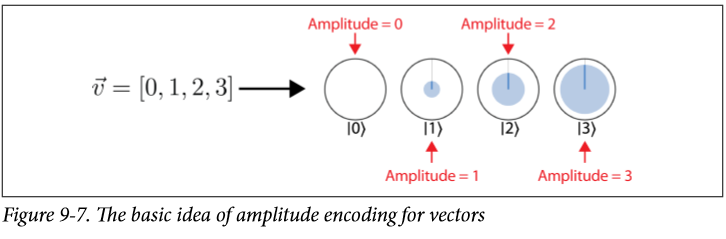• 因为概率需要归一化，所以他能表示的态也需要归一化

1. Deconstruct $H=H_{1}+\ldots H_{n}$ 把H分解成更容易模拟的hermitian（也就是更容易执行第二步）
2. Simulate components $U=\exp (-i H t)$ 操作只有unitary的操作，但是矩阵可以把他们联系到一起
3. Reconstruction 把得到的U乘起来，因为H是在指数上分解的，所以这里直接乘起来就可以

### 量子搜索Quantum Search (QS)

Phase Logic ，我们不仅可以改变他们的0或者1，还可以通过01来改变量子态的相位，就像这样：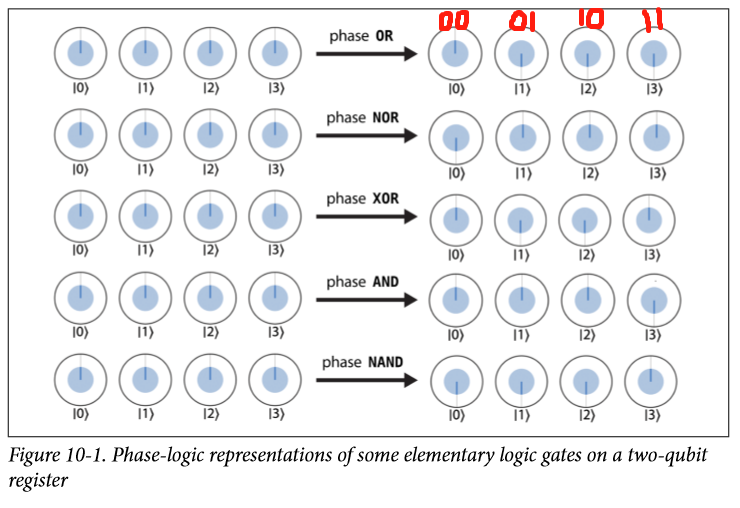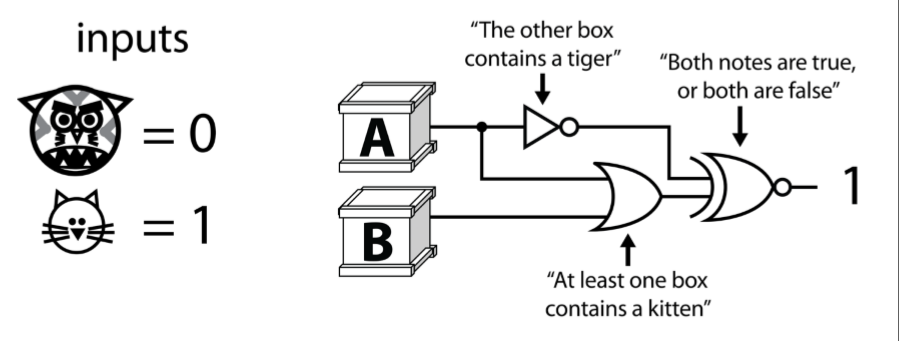这样，跑把四种可能性都跑一遍，输出为1就满足了逻辑。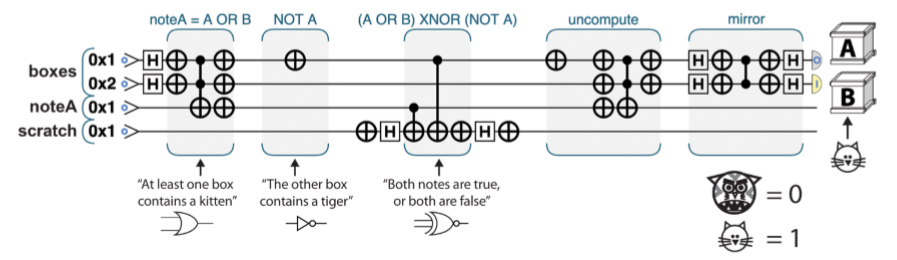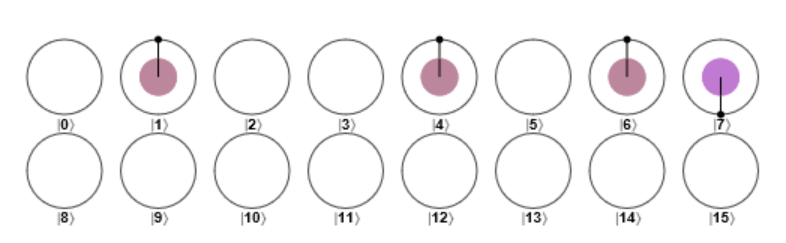Grover mirror 后：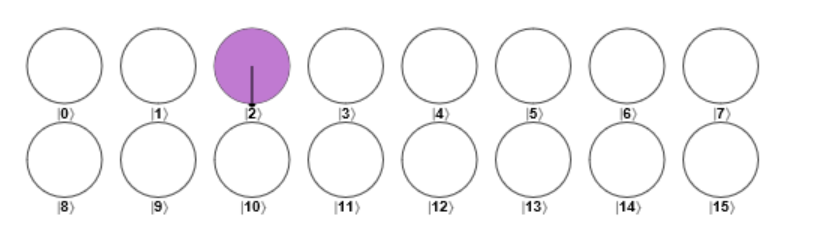### 量子超采样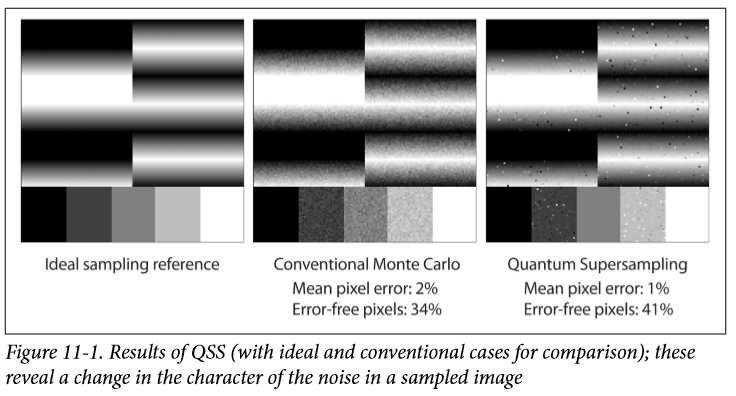### Shor算法

var N = 15;             // The number we're factoring
var precision_bits = 4; // See the text for a description of this
var coprime = 2;        // For this QPU implementation, this must be 2
var result = Shor(N, precision_bits, coprime);


• Step 1: Initialize QPU Registers
• Step 2: Expand into Quantum Superposition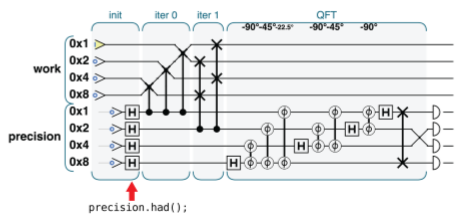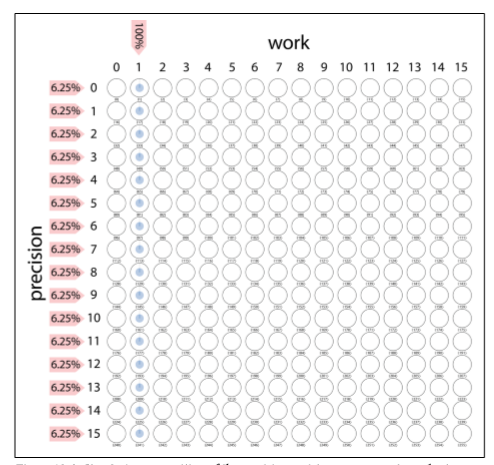• Step 3: Conditional Multiply-by-2

• Step 4: Conditional Multipy-by-4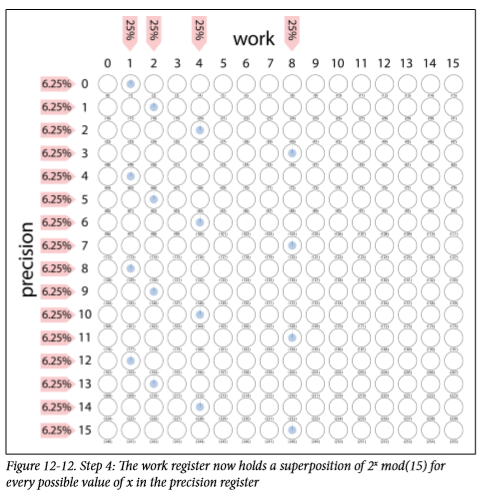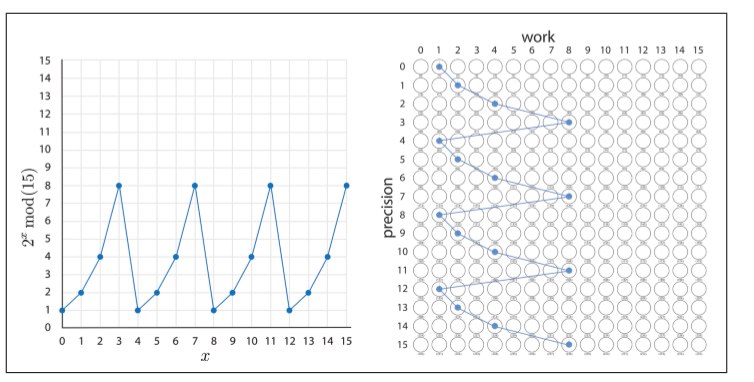• Step 5: Quantum Fourier Transform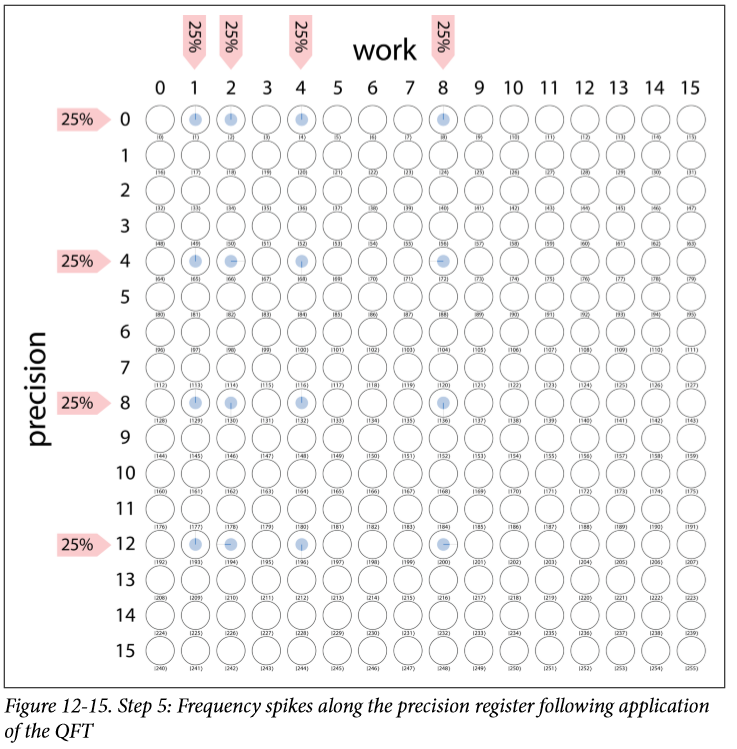• Step 6: Read the Quantum Result

• Step 7: Digital Logic
function ShorLogic(N, repeat_period, coprime) {    // Given the repeat period, find the actual factors
var ar2 = Math.pow(coprime, repeat_period / 2.0);
var factor1 = gcd(N, ar2 - 1);
var factor2 = gcd(N, ar2 + 1);
return [factor1, factor2];
}


• Step 8: Check the Result

### 量子机器学习

#### HHL解线性方程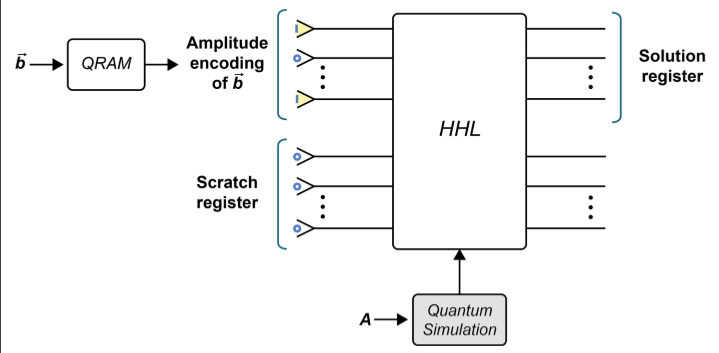Scratch register 也就是辅助比特包含由HHL中的各种基元使用的许多临时量子位，所有临时量子位均以“ 0”状态准备。因为HHL处理定点（或浮点）数据并且涉及非平凡的算术运算（例如取平方根），所以我们需要大量的临时qubit。即使最简单的情况，这也使得HHL难以仿真。

• 我们可能不需要$|x\rangle$的每个分量的值，而是他们平均值或者和
• 或者我们只想要比较一下看是否等于
• 或者$|x\rangle$只是程序下一部分的输入

HHL算法适合于求解由稀疏，条件良好的矩阵表示的线性方程组。

HHL的灵感来自特征值分解来获得矩阵逆的信息，一个栗子：

$$A=\left|\begin{array}{ll} 2 & 2 \ 2 & 3 \end{array}\right|, \quad \vec{z}=\left|\begin{array}{l} 1 \ 0 \end{array}\right|$$

A的特征向量为$v_1 = [−0.7882,0.615]$ and $v_2 = [−0.615, −0.788]$,对应的特征值为 λ1 = 0.438 and λ2 = 4.56， 那么，在特征基的表示下，我们可以把z改写成 [−0.788, −0.615]，因为$\vec{z}=-0.788 \vec{v}{1}-0.615 \vec{v}{2}$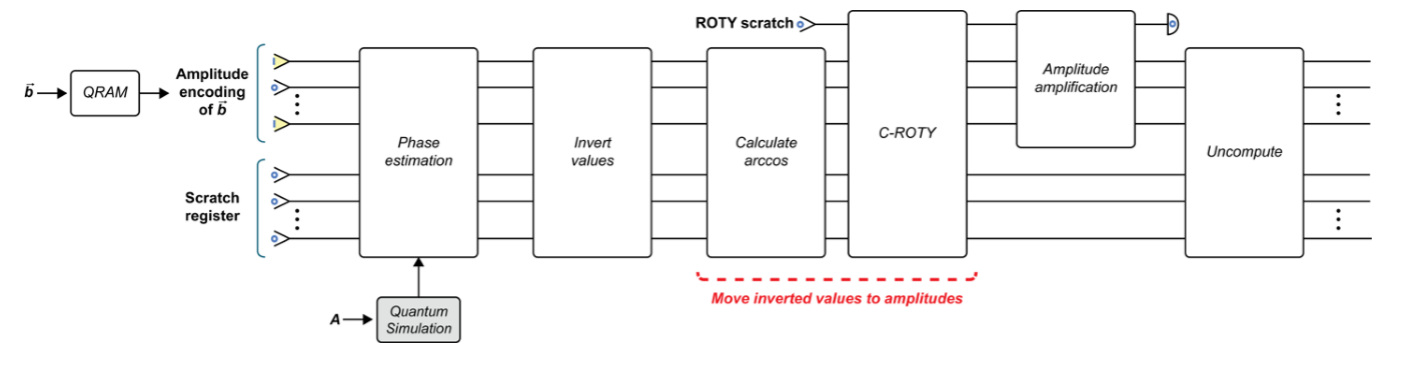1. Quantum simulation, QRAM, and phase estimation
2. Invert values
3. Move inverted values into amplitudes
4. Amplitude amplification
5. Uncompute

#### 量子主成分分析

• 将数据的协方差矩阵表示为QPU操作。
• 对此QPU操作执行相位估计，以确定其特征值。

• Quantum simulation with σ

协方差矩阵很少能满足量子模拟技术的稀疏性要求，因此我们需要一种不同的方法来找到σ的QPU运算表示。

• Input for phase estimation

• 用mini-SWAP来改变矩阵的稀疏性，具体见 https://arxiv.org/abs/1307.0401
• σ的密度算符表示正好是我们相位估计的输入，如果这样的话，输出就正好是编码了的特征值。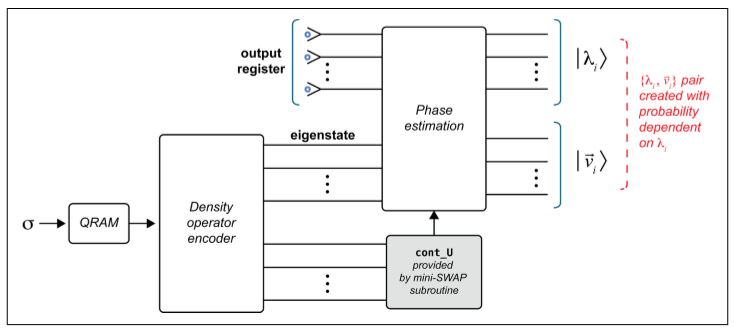0
0 收藏

### 作者的其它热门文章0 评论
0 收藏
0